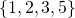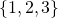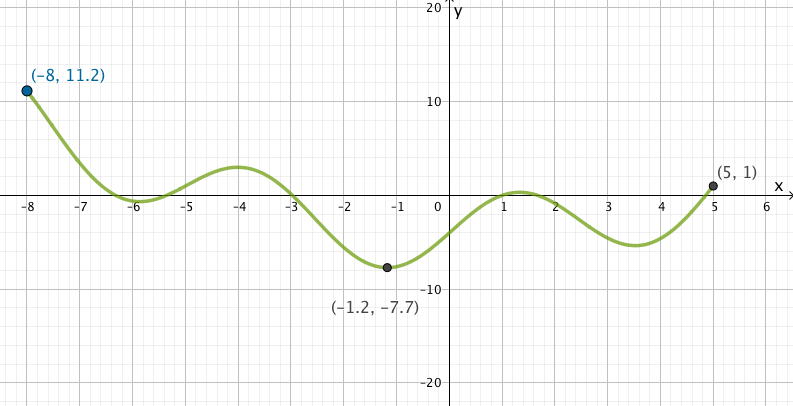# Domain and Range

A relation is a set of ordered pairs. In each pair, the first element comes from a set called the domain, the second from a set called the range.

Example 1

Domain:Range:Now letrepresent an element from the domain, andrepresent an element form the range. We can define the relation:is related toifis a member of the house of.

This generates the following relation:In this relation, there are six elements in the domain; four in the range and six ordered pairs in the relation.

A relation is a broad concept, and can have some meaning that is recognisable or can seem quite random.

Example 2It seems a little random, and it is. However it fits the definition of a set of ordered pairs, and so it is a relation. In this case, we have the following:

Domain:Range:Example 3

We can read the domain and range of a relation from a graph.On this graph, the following four points are plotted:The domain is therefore the set.

The range is the set. We need only mention the y value ‘2’ once, even though it appears more than once in the set of ordered pairs.

## Example 4

On this graph, we see a continuous curve. A continuous curve represents infinitely many points, so we can’t list them individually.

The domain and range are also infinite sets – they have a max and a min each, but inifinitely many points inbetween.

For the domain, we’re looking at the horizontal axis. In this case, theaxis. We’re looking for the minimum value ofand the maximum value ofincluded on the set of green points that makes up the curve.

For the range, we’re looking at the vertical axis. We’re looking for the minimum and the maximum value ofon the green curve.Domain:Range:Notice that we can read the domain from the endpoints, but the minimum and maximum values of y could appear anywhere in between the endpoints.

## Example 5

In some sports, when a ball hits the boundary line of the court it is considered ‘in’ (eg, soccer) in other sports it is considered ‘out’ (eg basketball). In math we use solid lines to show boundaries that are ‘in’ and we use dotted lines to show boundaries that are ‘out’.  (Example here). Similarly with endpoints, we use solid points to show endpoints that are ‘in’ and empty points to show endpoints that are ‘out.’ The symbols we use to differentiate between inclusion and exclusion are:(to include the boundary/endpoint); or(to exclude the boundary/endpoint).# Practice writing the domain and range

Use the red dynamic point to find the max/min x coordinates and y coordinates. Click the ‘trace button’ then move the red dynamic point to see the domain/range traced on the x, y axes. Write the domain and the range for each function. Solutions are on the pdf linked below the applet.